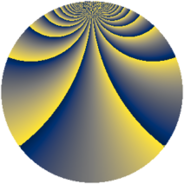# Properties

 Label 1815.2.biLevel $1815$ Weight $2$ Character orbit 1815.bi Rep. character $\chi_{1815}(43,\cdot)$ Character field $\Q(\zeta_{44})$ Dimension $2640$ Sturm bound $528$

# Related objects

## Defining parameters

 Level: $$N$$ $$=$$ $$1815 = 3 \cdot 5 \cdot 11^{2}$$ Weight: $$k$$ $$=$$ $$2$$ Character orbit: $$[\chi]$$ $$=$$ 1815.bi (of order $$44$$ and degree $$20$$) Character conductor: $$\operatorname{cond}(\chi)$$ $$=$$ $$605$$ Character field: $$\Q(\zeta_{44})$$ Sturm bound: $$528$$

## Dimensions

The following table gives the dimensions of various subspaces of $$M_{2}(1815, [\chi])$$.

Total New Old
Modular forms 5360 2640 2720
Cusp forms 5200 2640 2560
Eisenstein series 160 0 160

## Trace form

 $$2640q - 8q^{5} + O(q^{10})$$ $$2640q - 8q^{5} + 44q^{10} + 8q^{11} - 16q^{12} + 44q^{13} + 8q^{15} + 264q^{16} + 40q^{20} + 56q^{22} + 32q^{23} + 8q^{25} + 16q^{26} + 16q^{31} - 16q^{33} + 264q^{36} - 124q^{37} + 56q^{38} - 8q^{42} - 32q^{48} - 220q^{50} - 24q^{53} - 136q^{55} + 384q^{56} + 48q^{58} - 32q^{60} + 16q^{66} + 192q^{67} + 112q^{70} + 16q^{75} - 264q^{76} - 24q^{77} + 80q^{80} - 2640q^{81} + 48q^{82} + 176q^{85} - 64q^{86} - 104q^{88} - 16q^{91} + 320q^{92} + 48q^{93} - 88q^{97} + O(q^{100})$$

## Decomposition of $$S_{2}^{\mathrm{new}}(1815, [\chi])$$ into newform subspaces

The newforms in this space have not yet been added to the LMFDB.

## Decomposition of $$S_{2}^{\mathrm{old}}(1815, [\chi])$$ into lower level spaces

$$S_{2}^{\mathrm{old}}(1815, [\chi]) \cong$$ $$S_{2}^{\mathrm{new}}(605, [\chi])$$$$^{\oplus 2}$$